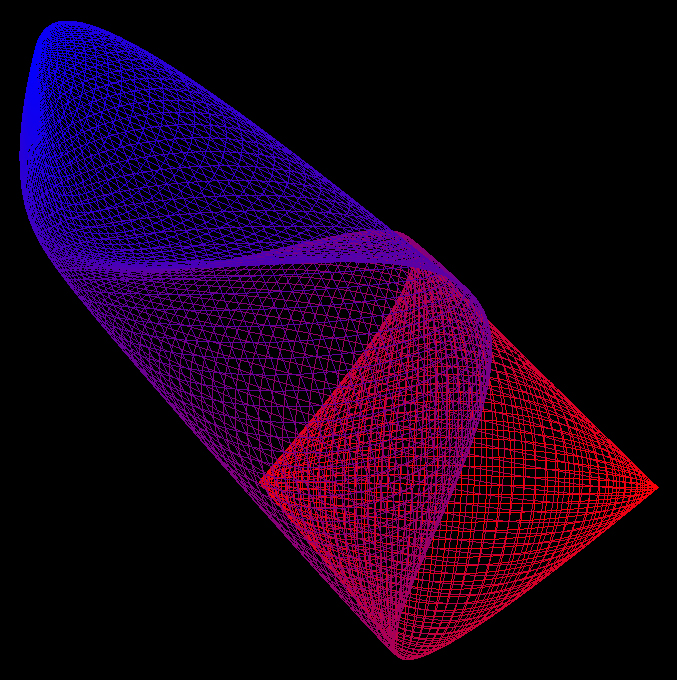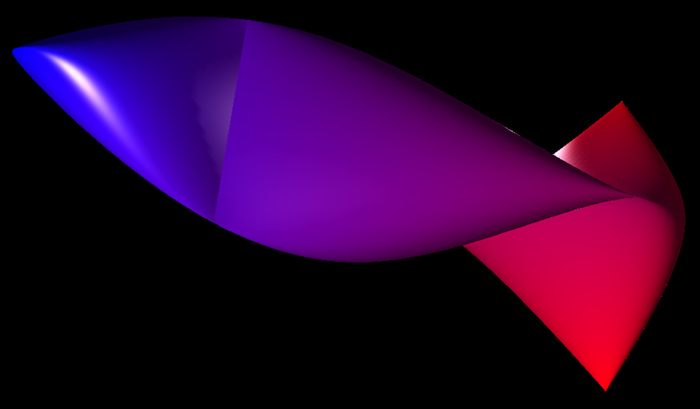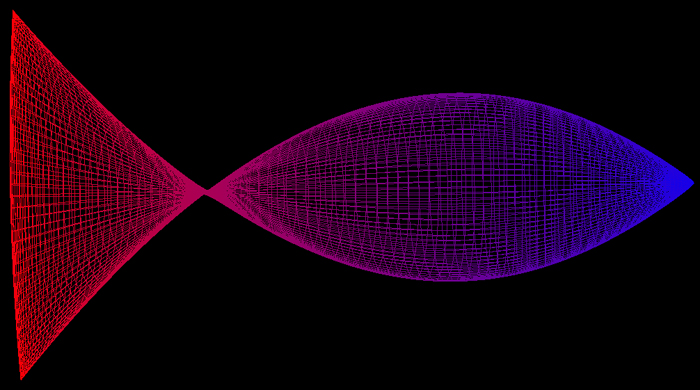# Fish Surface

By Roger Bagula
Graphics by Paul Bourke
October 2002
x = [ cos(u) - cos(2 u) ] cos(v) / 4
y = [ sin(u) - sin(2 u) ] sin(v) / 4
z = cos(u)
0 <= u <= pi, 0 <= v <= 2 pi